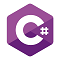# Snippets Zone#### FizzBuzz in C#

1235  25-June-2021

FizzBuzz in an important interview question asked by several interviewers for checking the logic skills of the developer.

FizzBuzz in an important interview question asked by several interviewers for checking the logic skills of the developer. During the interview, the interviewer can ask to print the number from 1 to 100 in such a manner when a number which is multiple of three, print “Fizz”  on the console/terminal, if multiple of five then print “Buzz”. If the number is multiple of three as well five, then print “FizzBuzz” in that place. Below is the sample code snippet which can be used for reference purpose.

``````static void Main(string[] args)
{
for (int i = 1; i <= 100; i++)
{
if (i % 3 == 0 && i % 5 == 0)
{
Console.WriteLine("FizzBuzz");
}
else if (i % 3 == 0)
{
Console.WriteLine("Fizz");
}
else if (i % 5 == 0)
{
Console.WriteLine("Buzz");
}
else
{
Console.WriteLine(i);
}
}
}``````

Another code snippet is mentioned below:

``````static void Main(string[] args)
{
for (int i = 1; i <= 100; i++)
{
string str = "";
if (i % 3 == 0)
{
str += "Fizz";
}
if (i % 5 == 0)
{
str += "Buzz";
}
if (str.Length == 0)
{
str = i.ToString();
}
Console.WriteLine(str);

}
}``````